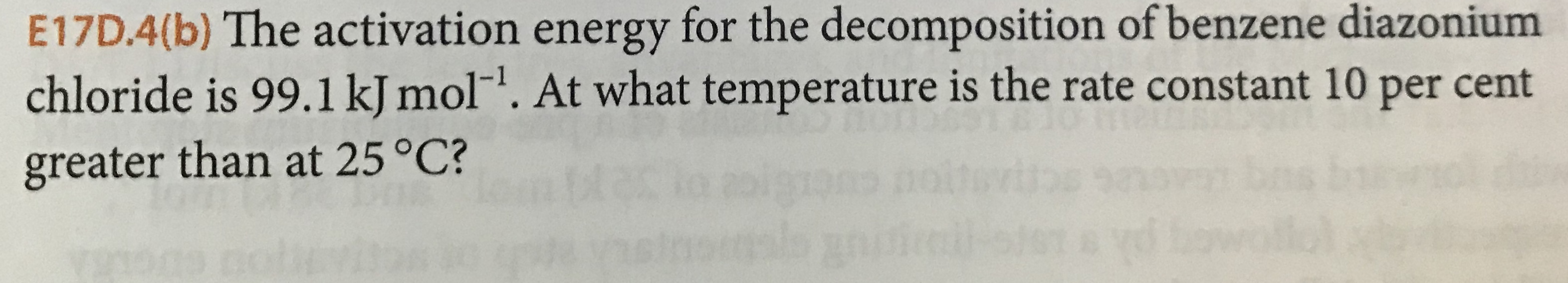# E17D.4(b) The activation energy for the decomposition ofbenzene diazoniumchloride is 99.1 kJ mol". At what temperature is the rate constant 10 per centgreater than at 25 °C?16

Question
1 viewshelp_outlineImage TranscriptioncloseE17D.4(b) The activation energy for the decomposition ofbenzene diazonium chloride is 99.1 kJ mol". At what temperature is the rate constant 10 per cent greater than at 25 °C? 16 fullscreen
check_circle

Step 1

The activation energy at 25˚C is 99.1 kJ/mol.

Let k1 is the rate constant at 25˚C and k2 at T2.

The conversion of temperature in Celsius to Kelvin is done by the formula.

Step 2

Therefore, the temperature (T1) is 289 K.

Similarly, the conversion of 99.1 kJ/mol into J/mol is as follows:

Step 3

It is given that rate constant (k2) is 10% greater than k1, which means k2 = 1.10 k1.

The form of Arrhenius e...

### Want to see the full answer?

See Solution

#### Want to see this answer and more?

Solutions are written by subject experts who are available 24/7. Questions are typically answered within 1 hour.*

See Solution
*Response times may vary by subject and question.
Tagged in

### Chemical Kinetics